true
true

Vishaka B.

Old Madiwala, Bangalore, India - 560068# Vishaka B.

## Home Tutor

Old Madiwala, Bangalore, India - 560068.

1 Student taught

4.2

UrbanPro Rating

Details verified of Vishaka B.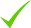IdentityEducation

Know how UrbanPro verifies Tutor details

Identity is verified based on matching the details uploaded by the Tutor with government databases.

## Overview

I can teach each and every topics very easily and make it stronger for the students.

## Languages Spoken

English Proficient

Hindi Proficient

## Education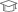visveswarya technological university 2017

Bachelor of Engineering (B.E.)

Old Madiwala, Bangalore, India - 560068

## Verified Info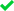Phone VerifiedEmail VerifiedFacebook Verified

Report this Profile

Is this listing inaccurate or duplicate? Any other problem?

Type the letters as shown below *

Please enter the letters as show below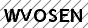Nursery-KG Tuition

Class LocationStudent's HomeTutor's Home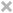Online (video chat via skype, google hangout etc)

Years of Experience in Nursery-KG Tuition

3

Subject

Mathematics, Drawing, English, EVS

Taught in School or College

No

## Reviews

this is test message this is test message this is test message this is test message this is test message this is test message this is test message

No Reviews yet! Be the first one to Review

## FAQs

1. Which Nursery-KG subjects do you tutor for?

Mathematics, Drawing, English and others

2. Do you have any prior teaching experience?

No

3. Which classes do you teach?

I teach Class 6 Tuition, Class I-V Tuition and Nursery-KG Tuition Classes.

4. Do you provide a demo class?

Yes, I provide a free demo class.

5. How many years of experience do you have?

I have been teaching for 3 years.

## Answers by Vishaka B. (651)

Answered on 09/10/2019 CBSE/Class 11/Science/Chemistry/States of Matter/NCERT Solutions/Exercise 5

Explain the physical significance of van der Waals parameters. ...more
Explain the physical significance of van der Waals parameters.

Physical significance of ‘a’: ‘a’ is a measure of the magnitude of intermolecular forces within a gas. Physical significance of ‘b’: ‘b’ is a measure of the volume of a gas molecule.
Dislike Bookmark

Answered on 09/10/2019 CBSE/Class 11/Science/Chemistry/States of Matter/NCERT Solutions/Exercise 5

Critical temperature for carbon dioxide and methane are 31.1 °C and –81.9 °C respectively.... ...more
Critical temperature for carbon dioxide and methane are 31.1 °C and –81.9 °C respectively. Which of these has stronger intermolecular forces and why?

Higher is the critical temperature of a gas, easier is its liquefaction. This means that the intermolecular forces of attraction between the molecules of a gas are directly proportional to its critical temperature. Hence, intermolecular forces of attraction are stronger in the case of CO2. ...more

Higher is the critical temperature of a gas, easier is its liquefaction. This means that the intermolecular forces of attraction between the molecules of a gas are directly proportional to its critical temperature. Hence, intermolecular forces of attraction are stronger in the case of CO2.

Dislike Bookmark

Answered on 09/10/2019 CBSE/Class 11/Science/Chemistry/States of Matter/NCERT Solutions/Exercise 5

In terms of Charles’ law explain why –273 °C is the lowest possible temperature

Charles’ law states that at constant pressure, the volume of a fixed mass of gas is directly proportional to its absolute temperature. It was found that for all gases (at any given pressure), the plots of volume vs. temperature (in °C) is a straight line. If this line is extended to zero volume,... ...more

Charles’ law states that at constant pressure, the volume of a fixed mass of gas is directly proportional to its absolute temperature.It was found that for all gases (at any given pressure), the plots of volume vs. temperature (in °C) is a straight line. If this line is extended to zero volume, then it intersects the temperature-axis at – 273°C. In other words, the volume of any gas at –273°C is zero. This is because all gases get liquefied before reaching a temperature of – 273°C. Hence, it can be concluded that – 273°C is the lowest possible temperature.

Dislike Bookmark

Answered on 09/10/2019 CBSE/Class 11/Science/Chemistry/States of Matter/NCERT Solutions/Exercise 5

What would be the SI unit for the quantity pV 2T 2/n ?

The SI unit for pressure, p is Nm–2. The SI unit for volume, V is m3. The SI unit for temperature, T is K. The SI unit for the number of moles, n is mol. Therefore, the SI unit for quantity is given by, ...more

The SI unit for pressure, p is Nm–2.

The SI unit for volume, V is m3.

The SI unit for temperature, T is K.

The SI unit for the number of moles, n is mol.

Therefore, the SI unit for quantityis given by,Dislike Bookmark

Answered on 09/10/2019 CBSE/Class 11/Science/Chemistry/States of Matter/NCERT Solutions/Exercise 5

A mixture of dihydrogen and dioxygen at one bar pressure contains 20% by weight of dihydrogen. Calculate... ...more
A mixture of dihydrogen and dioxygen at one bar pressure contains 20% by weight of dihydrogen. Calculate the partial pressure of dihydrogen.

Let the weight of dihydrogen be 20 g and the weight of dioxygen be 80 g. Then, the number of moles of dihydrogen, and the number of moles of dioxygen, . Given, Total pressure of the mixture, ptotal = 1 bar Then, partial pressure of dihydrogen, Hence, the partial pressure of dihydrogen is. ...more

Let the weight of dihydrogen be 20 g and the weight of dioxygen be 80 g.

Then, the number of moles of dihydrogen,and the number of moles of dioxygen,.

Given,

Total pressure of the mixture, ptotal = 1 bar

Then, partial pressure of dihydrogen,Hence, the partial pressure of dihydrogen is.

Dislike Bookmark
Nursery-KG Tuition 4.2

Class LocationStudent's HomeTutor's HomeOnline (video chat via skype, google hangout etc)

Years of Experience in Nursery-KG Tuition

3

Subject

Mathematics, Drawing, English, EVS

Taught in School or College

No

Class I-V Tuition 4.2

Class LocationStudent's HomeTutor's HomeOnline (video chat via skype, google hangout etc)

Years of Experience in Class I-V Tuition

3

Fees

₹ 300 per hour

Board

State, ICSE, IGCSE, CBSE

CBSE Subjects taught

Mathematics, EVS, Hindi, Science, English

ICSE Subjects taught

Social Studies, Hindi, EVS, Mathematics

IGCSE Subjects taught

English, Mathematics

Taught in School or College

No

State Syllabus Subjects taught

Mathematics, EVS, Science, English, Hindi

Class 6 Tuition 4.2

Class LocationStudent's HomeTutor's HomeOnline (video chat via skype, google hangout etc)

Years of Experience in Class 6 Tuition

5

Board

ICSE, CBSE

CBSE Subjects taught

Social Science, Mathematics, Science, Hindi, EVS, English

ICSE Subjects taught

Mathematics, English, History, EVS

Taught in School or College

No

this is test message this is test message this is test message this is test message this is test message this is test message this is test message

No Reviews yet! Be the first one to Review

## Answers by Vishaka B. (651)

Answered on 09/10/2019 CBSE/Class 11/Science/Chemistry/States of Matter/NCERT Solutions/Exercise 5

Explain the physical significance of van der Waals parameters. ...more
Explain the physical significance of van der Waals parameters.

Physical significance of ‘a’: ‘a’ is a measure of the magnitude of intermolecular forces within a gas. Physical significance of ‘b’: ‘b’ is a measure of the volume of a gas molecule.
Dislike Bookmark

Answered on 09/10/2019 CBSE/Class 11/Science/Chemistry/States of Matter/NCERT Solutions/Exercise 5

Critical temperature for carbon dioxide and methane are 31.1 °C and –81.9 °C respectively.... ...more
Critical temperature for carbon dioxide and methane are 31.1 °C and –81.9 °C respectively. Which of these has stronger intermolecular forces and why?

Higher is the critical temperature of a gas, easier is its liquefaction. This means that the intermolecular forces of attraction between the molecules of a gas are directly proportional to its critical temperature. Hence, intermolecular forces of attraction are stronger in the case of CO2. ...more

Higher is the critical temperature of a gas, easier is its liquefaction. This means that the intermolecular forces of attraction between the molecules of a gas are directly proportional to its critical temperature. Hence, intermolecular forces of attraction are stronger in the case of CO2.

Dislike Bookmark

Answered on 09/10/2019 CBSE/Class 11/Science/Chemistry/States of Matter/NCERT Solutions/Exercise 5

In terms of Charles’ law explain why –273 °C is the lowest possible temperature

Charles’ law states that at constant pressure, the volume of a fixed mass of gas is directly proportional to its absolute temperature. It was found that for all gases (at any given pressure), the plots of volume vs. temperature (in °C) is a straight line. If this line is extended to zero volume,... ...more

Charles’ law states that at constant pressure, the volume of a fixed mass of gas is directly proportional to its absolute temperature.It was found that for all gases (at any given pressure), the plots of volume vs. temperature (in °C) is a straight line. If this line is extended to zero volume, then it intersects the temperature-axis at – 273°C. In other words, the volume of any gas at –273°C is zero. This is because all gases get liquefied before reaching a temperature of – 273°C. Hence, it can be concluded that – 273°C is the lowest possible temperature.

Dislike Bookmark

Answered on 09/10/2019 CBSE/Class 11/Science/Chemistry/States of Matter/NCERT Solutions/Exercise 5

What would be the SI unit for the quantity pV 2T 2/n ?

The SI unit for pressure, p is Nm–2. The SI unit for volume, V is m3. The SI unit for temperature, T is K. The SI unit for the number of moles, n is mol. Therefore, the SI unit for quantity is given by, ...more

The SI unit for pressure, p is Nm–2.

The SI unit for volume, V is m3.

The SI unit for temperature, T is K.

The SI unit for the number of moles, n is mol.

Therefore, the SI unit for quantityis given by,Dislike Bookmark

Answered on 09/10/2019 CBSE/Class 11/Science/Chemistry/States of Matter/NCERT Solutions/Exercise 5

A mixture of dihydrogen and dioxygen at one bar pressure contains 20% by weight of dihydrogen. Calculate... ...more
A mixture of dihydrogen and dioxygen at one bar pressure contains 20% by weight of dihydrogen. Calculate the partial pressure of dihydrogen.

Let the weight of dihydrogen be 20 g and the weight of dioxygen be 80 g. Then, the number of moles of dihydrogen, and the number of moles of dioxygen, . Given, Total pressure of the mixture, ptotal = 1 bar Then, partial pressure of dihydrogen, Hence, the partial pressure of dihydrogen is. ...more

Let the weight of dihydrogen be 20 g and the weight of dioxygen be 80 g.

Then, the number of moles of dihydrogen,and the number of moles of dioxygen,.

Given,

Total pressure of the mixture, ptotal = 1 bar

Then, partial pressure of dihydrogen,Hence, the partial pressure of dihydrogen is.

Dislike Bookmark

Vishaka B. describes herself as Home Tutor. She conducts classes in Class 6 Tuition, Class I-V Tuition and Nursery-KG Tuition. Vishaka is located in Old Madiwala, Bangalore. Vishaka takes at students Home and Regular Classes- at her Home. She has 5 years of teaching experience . Vishaka has completed Bachelor of Engineering (B.E.) from visveswarya technological university in 2017. She is well versed in English and Hindi.

•• Want to learn from Vishaka B.?

• Contact Now
X

Recommended Profiles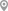JP Nagar 7th Phase, BangaloreMadras Bombay Trunk Rd, BangaloreRajaji Nagar, BangaloreHBR Layout, BangaloreJayanagar, BangaloreVarthur, Bangalore

X

Let us shortlist and give the best tutors and institutes.

or

Send Enquiry to Vishaka B.

Let Vishaka B. know you are interested in their class